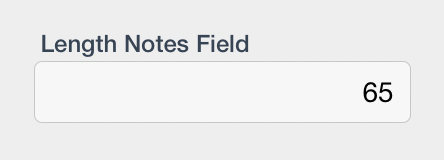DESCRIPTION: The length() function will return the number of characters in a string. If the input is a null value, the formula will return 0. When counting characters using this function, remember that a blank between words will be counted as a character.

SYNTAX
length(String)

WHERE

• length, in lowercase initiates the function
• String is a single (not an array) Text element

Things to keep in mind when constructing this function:
1. The key word of the function is case sensitive and must be entered in all lower case
2. The String element is required
3. The result of the formula is a number
4. You will receive an error in the formula if the input is not a Text element
5. To count the number of characters in a non-text string, first convert the string to text using the text() function.

EXAMPLE
length(“Nioxus is great!”) = 16
length(text(1000.00)) = 7

USAGE
You have created a table that contains a multi-line text field and that field has a content limit of of 250 characters as defined in the Max Length parameter of the field itself. During data entry, you want to provide the user with a count indicating the number of characters remaining available to be added to the field. Using the length function, you can create a calculation as follows:

250-length(‘Multi Line Text Field’)

The result of the calculation will be the number of characters in the field subtracted from 250 resulting in a display of the number of characters that may still be added to the field.

Here’s the code:

length(Notes)

You’ll then get a field that looks like this:Revision: 2